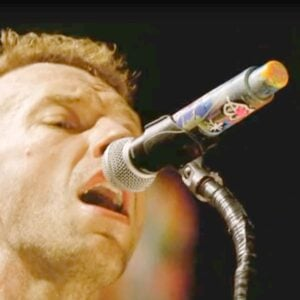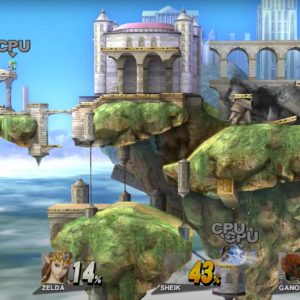# Harus Terpisah

10 September 2020
2
• ##### TYPE
0
(0)

8 9 0 8 0 0 0 q 9 8 9 9 9 w 8
8 9 0 0 q w q 0 q w e r e 0
8 r e r t y w y y y u t
r e w q 0 9 9 e w 0 E e w e w ewq
q W w q w 0 9 0 q w q q e t y t w

w w t y t r t w w w t y u i u y t
t t r t y u t e w e t i u y u
w w t y t y u u y t y u i i u y y t

e u u t e q 0 9 0 w w 0
e w 0 8 e w w q w 8 w q 0 9 8
8 9 0 0 q w q 0 q w e r e 0

8 r e r t y w y y y u t
r e w q e t y o u

w w t y t r t w w w t y u i u y t
t t r t y u t e w e t i u y u

Rate This Music Sheet:

Average rating 0 / 5. Vote count: 0

No votes so far! Be the first to rate this music sheet.

Thank you for rating this song!

If you have any specific feedback about how to improve this music sheet, please submit this in the box below.

Harus Terpisah is a song by Cakra Khan. Use your computer keyboard to play Harus Terpisah music sheet on Virtual Piano. This is an Easy song and requires practice. The song Harus Terpisah is classified in the genre of Pop on Virtual Piano. You can also find other similar songs using Sad, Indonesia.

## Related

•[9d] p I d p I d p
[6s] p u s p u s p
[6s] p u s p u s p
[0a] o u a o u a |
d d d dasa p |
s sss pap o y |
d d d dasa p |
s sss pap o y

Level: 4
Length: 00:25
Easy

#### Coldplay

•yEw yEw yEw YEw YEw Yew uEw uEw uEw YEw YEw Yew 555556655 555556655 pPd DPDf PfD hgD
Level: 3
Easy
##### Hyrule Temple (Super Smash Bros)
•[tqpe] s d h d s [tqpe] s d h d s p s d h [ywo0] s d h d s [ywo0] s d h d s o s d h [utpe] s f j f s [utpe] s f j f s p s f j [ywta] s d h d s [ywta] s d h d s [ywa0] s d h [tqpe] s d h d s [tqpe] s d h d s p s d h [ywo0] s d h d s [ywo0] s d h d s o s d h [utpe] s f j f s [utpe] s f j f s p s f j [ywta] s d h d s [ywta] s d h d s [ywa0] s d h [tqpe] s d h d s [tqpe] s d h d s p s d h [ywo0] s d h d s [ywo0] s d h d s o s d h [utpe] s f j f s [utpe] s f j f s p s f j [ywta] s d h d s [ywta] s d h d s [ywa0] s d h [tqpe] s d h d s [tqpe] s d h d s p s d h [ywo0] s d h d s [ywo0] s d h d s o s d h [utpe] s f j f s [utpe] s f j f s p s f j [ywta] s d h d s [ywta] s d h d s [ywa0] s d h [tqpe] s d h d s [tqpe] s d h d s p s d h [ywo0] s d h d s [ywo0] s d h d s o s d h [utpe] s f j f s [utpe] s f j f s p s f j [ywta] s d h d s [ywta] s d h d s [ywa0] s d h [tqpe] s d h d s [tqpe] s d h d s p s d h [ywo0] s d h d s [ywo0] s d h d s o s d h [utpe] s f j f s [utpe] s f j f s p s f j [ywta] s d h d s [ywta] s d h d s [ywa0] s d| [tqpe] s d h d s [tqpe] s d h d s p s d h [ywo0] s d h d s [ywo0] s d h d s o s d h [utpe] s f j f s [utpe] s f j f s p s f j [ywta] s d h d s [ywta] s d h d s [ywa0] s d h [tqpe] s d h d s [tqpe] s d h d s p s d h [ywo0] s d h d s [ywo0] s d h d s o s d h [utpe] s f j f s [utpe] s f j f s p s f j [ywta] s d h d s [ywta] s d h
Level: 6
Length: 01:56
Intermediate